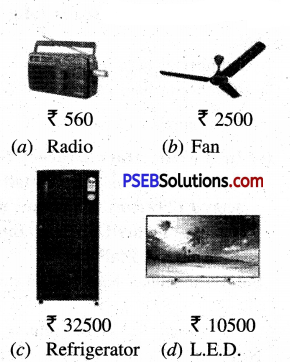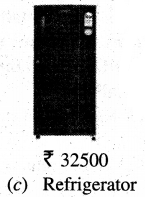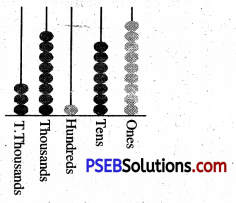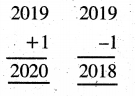# PSEB 5th Class Maths MCQ Chapter 1 Numbers

Punjab State Board PSEB 5th Class Maths Book Solutions Chapter 1 Numbers MCQ Questions and Answers.

## PSEB 5th Class Maths Chapter 1 Numbers MCQ Questions

Tick (✓) the right answer :

Question 1.
Write the successor of the greatest 5-digit number.
(a) 99999
(b) 10000
(c) 100000
(d) 9999.
(c) 100000

Question 2.
How many 2-digits numbers are there ?
(a) 99
(b) 90
(c) 100
(d) 89
(b) 90Question 3.
How many 5-digits numbers are there ?
(a) 99999
(b) 9000
(c) 10000
(d) 90000.
(d) 90000.

Question 4.
Smallest 5-digits number using digits 4, 6, 8, 9, 0 is :
(a) 46890
(b) 04689
(c) 98640
(d) 40689.
(d) 40689.

Question 5.
Fifty nine thousand fifty nine = ______
(a) 59590
(b) 5959
(c) 59059
(d) 59509.
(c) 59059

Question 6.
Choose the place value of 6 in 26573.
(a) 60000
(b) 6000
(c) 6
(d) 60.
(b) 6000

Question 7.
Standard form of 20000 + 5000 + 30 + 4 is :
(a) 25304
(b) 25034
(c) 20534
(d) 25043.
(b) 25034

Question 8.
Greatest 5-digits number by using digits 7, 8, 6, 7, 9 :
(a) 67879
(b) 98767
(c) 98776
(d) 98677.
(c) 98776

Question 9.
Out of the following numbers, which number has the place value of 8 as 8000 ?
(a) 35832
(b) 43248
(c) 54682
(d) 48054.
(d) 48054.Question 10.
Write 48 is Roman Numerals.
(a) LVIII
(b) LXVIII
(c) XLVHI
(d) XVIIIL.
(c) XLVHI

Question 11.
Write 85 in Roman Numerals.
(a) LXXV
(b) XXCV
(c) XVC
(d) LXXXV.
(d) LXXXV.

Question 12.
Which is predecessor of 10000 ?
(a) 9999
(b) 999
(c) 99999
(d) 1000.
(a) 9999

Question 13.
Roman numeral of 94 is :
(a) CVI
(b) XCVI
(c) XCIV
(d) XICV.
(c) XCIV

Question 14.
Choose the correct numeral using I, X, L, V.
(a) XILV
(b) XLVI
(c) XVIL
(d) VXIL.
(b) XLVI

Question 15.
Greatest 5-digits number by using digits 1, 0, 3 is :
(a) 11103
(b) 10333
(c) 33310
(d) 10003.
(c) 33310

Question 16.
Smallest 4-digits number using digits 9, 8, 0 :
(a) 9800
(b) 9008
(c) 8090
(d) 8009.
(d) 8009.

Question 17.
Round off 758 to the nearest tens is :
(a) 750
(b) 760
(c) 800
(d) 700
(b) 760

Question 18.
Round off 8978 to the nearest tens is :
(a) 8980
(b) 9000
(c) 8970
(d) 8900.
(a) 8980

Question 19.
Round off 69684 to the nearest thousands is :
(a) 69000
(b) 69700
(c) 79000
(d) 70000.
(d) 70000.

Question 20.
If a number has to be rounded off to the nearest ten thousands then which place of the digit will be taken ?
(a) Tens
(b) Hundreds
(c) Thousands
(d) Ten Thousands.
(c) Thousands

Question 21.
Place value of 0 in 50358 is :
(a) 10000
(b) 100
(c) 1000
(d) 0.
(d) 0.

Question 22.
Which symbols are not repeated in Roman system ?
(a) L, X
(b) L, V
(c) X, I
(d) L, I.
(b) L, VQuestion 23.
How many digits are there in one lakh ?
(a) 5
(b) 6
(c) 4
(d) 7.
(b) 6

Question 24.
How many thousands are there in one lakh ?
(a) 10
(b) 100
(c) 1000
(d) 10000.
(b) 100

Question 25.
How many maximum number of beads can be inserted in abacus ?
(a) 1
(b) 10
(c) 0
(d) 9.
(d) 9.

Question 26.
In the given figure which object has maximum cost price ?Question 27.
Write the number by looking at abascus.(a) 8179
(b) 38179
(c) 3879
(d) 97183.